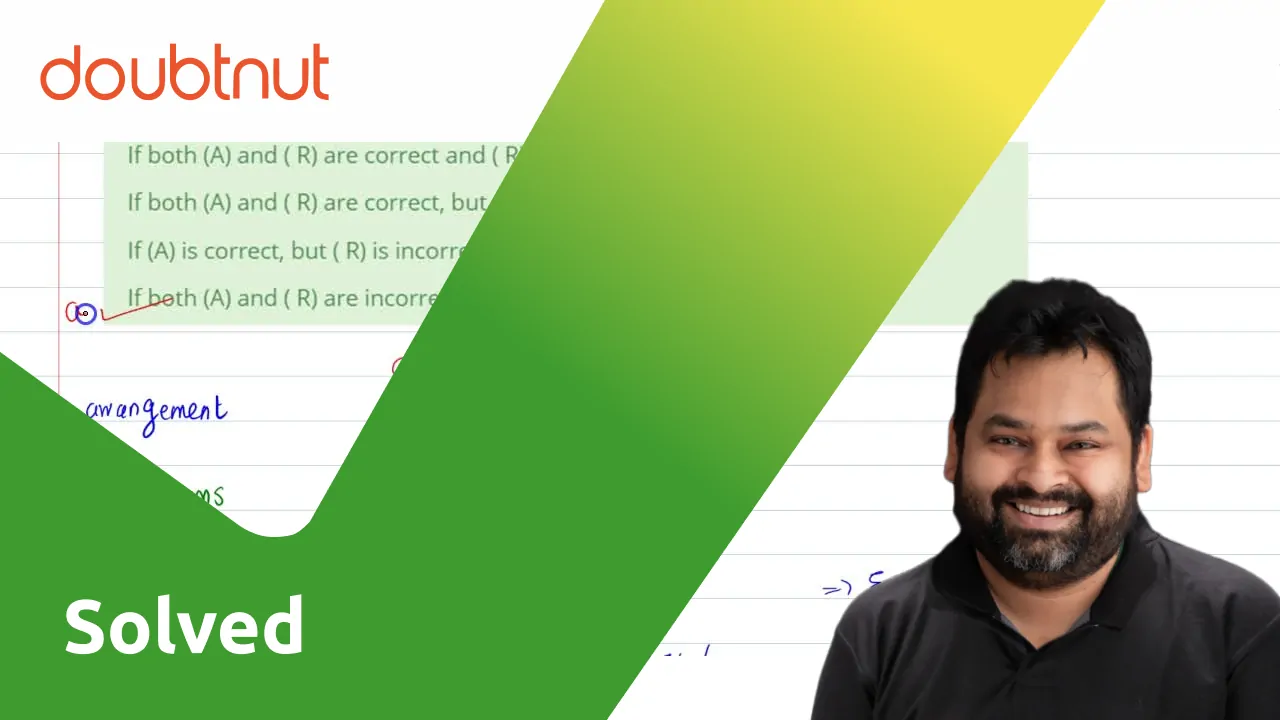Doubtnut App - Get 50 Lakh+ video solutions better than GoogleInstall Now

# Assertion : Hexagonal close packing is more closely packed than cubic close packing. Reason : Hexagonal close packing has a coordination number of 12 whereas cubic close packing has a coordination number of 8.Solution in Malayalam

A

If both (A) and ( R) are correct and ( R) is the correct explanation of(A).

B

If both (A) and ( R) are correct, but ( R) is not the correct explanation of (A).

C

If (A) is correct, but ( R) is incorrect.

D

If both (A) and ( R) are incorrect.

Text Solution
Verified by Experts

## Hexagonal close packing and cubic close packing are equally close packed (space occupied=74%). Both have a coordination number of 12.